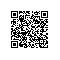# Python中典型内建函数的用法

abs(x)

all(x)

any(x)

ascii(x)

1. print(ascii('中文'))# '\u4e2d\u6587'

2. print('\u6587') # 文

bin(x)

bool(x)

bytearray()

1. print(bytearray('ok', 'utf-16'))

2. # bytearray(b'\xff\xfeo\x00k\x00')

3. print(bytearray(3))

4. # bytearray(b'\x00\x00\x00')

5. print(bytearray([1, 2, 200]))

6. # bytearray(b'\x01\x02\xc8')

bytes()

callable(x)

chr(x)

class method()

1. class C():

2. var = 1

3. @classmethod

4. def f(cls, arg1, arg2, ...):

5. cls.var = arg1

6. instance = cls()

compile(source, filename, mode)

1) 如果代码只是表达式，例如 2*3, 则mode = ‘eval’
2) 如果代码是交互式语句，例如a = input(),则mode = ‘single’
3) 其余情况，一般mode = ‘exec’

complex(x)

1. print(type(complex())) # <class 'complex'>

2. print(complex(1)) # (1+0j)

3. print(complex('2+1j')) # (2+1j)

delattr(x, name)Python中文社区使用钉钉扫一扫加入圈子
+ 订阅